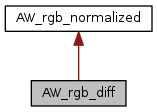ARB
AW_rgb_diff Class Reference

`#include <aw_rgb.hxx>`

Inheritance diagram for AW_rgb_diff:[legend]
Collaboration diagram for AW_rgb_diff:[legend]

## Public Member Functions

AW_rgb_diff (float red, float green, float blue)

float r () const

float g () const

float b () const

AW_rgb_diff abs () const

## Detailed Description

Definition at line 106 of file aw_rgb.hxx.

## Constructor & Destructor Documentation

 AW_rgb_diff::AW_rgb_diff ( float red, float green, float blue )
inline

Definition at line 108 of file aw_rgb.hxx.

Referenced by abs().

## Member Function Documentation

 float AW_rgb_diff::r ( ) const
inline

Definition at line 110 of file aw_rgb.hxx.

References AW_rgb_normalized::r().

Referenced by abs(), colorDistSquare(), max(), operator*(), and operator+().

 float AW_rgb_diff::g ( ) const
inline

Definition at line 111 of file aw_rgb.hxx.

References AW_rgb_normalized::g().

Referenced by abs(), colorDistSquare(), max(), operator*(), and operator+().

 float AW_rgb_diff::b ( ) const
inline

Definition at line 112 of file aw_rgb.hxx.

References AW_rgb_normalized::b().

Referenced by abs(), colorDistSquare(), max(), operator*(), and operator+().

 AW_rgb_diff AW_rgb_diff::abs ( ) const
inline

Definition at line 114 of file aw_rgb.hxx.

References AW_rgb_diff(), b(), g(), and r().

The documentation for this class was generated from the following file: